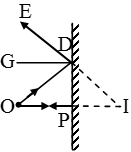# Physics

### Chapter : 3. Light Reflection

#### Reflection From The Plane Mirror

Reflection From The Plane Mirror
Relation between the distances of the object and the image from the plane mirror is that they are equal.
To verify this, consider the geometrical construction shown in figure. Rays OP and OD, starting from the object O, fall on the mirror. The ray OP is perpendicular to the mirror and hence, reflects back along PO. The incident ray OD and the reflected ray DE make equal angles with the normal DG. The two reflected rays when produced backwards meet at I, producing a virtual image there.Now, ∠EDG = ∠DIO (DG || IO)
∠EDG = ∠GDO (law of reflection), and
∠GDO = ∠DOI (DG || IO).
Hence, ∠DIO = ∠DOI
∴ OD = DI
Now OP2 = OD2 – DP2 and
PI2 = DI2 – DP2
From (i), since OD = DI, OP2 = PI2 or OP = PI.
So, in the case of a plane mirror, the image is formed as far behind the mirror at the same distance as the object is in front of it.